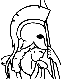#Convex Optimization Theory Table of Contents:

1. Basic Concepts of Convex Analysis

1. Convex Sets and Functions
1. Convex Functions
2. Closedness and Semicontinuity
3. Operations with Convex Functions
4. Characterizations of Differentiable Convex Functions
2. Convex and Affine Hulls
3. Relative Interior and Closure
1. Calculus of Relative Interiors and Closures
2. Continuity of Convex Functions
3. Closures of Functions
4. Recession Cones
1. Directions of Recession of a Convex Function
2. Nonemptiness of Intersections of Closed Sets
3. Closedness Under Linear Transformations
5. Hyperplanes
1. Hyperplane Separation
2. Proper Hyperplane Separation
3. Nonvertical Hyperplane Separation
6. Conjugate Functions
7. Summary

2. Basic Concepts of Polyhedral Convexity

1. Extreme Points
2. Polar Cones
3. Polyhedral Sets and Functions
1. Polyhedral Cones and Farkas' Lemma
2. Structure of Polyhedral Sets
3. Polyhedral Functions
4. Polyhedral Aspects of Optimization

3. Basic Concepts of Convex Optimization

1. Constrained Optimization
2. Existence of Optimal Solutions
3. Partial Minimization of Convex Functions
4. Saddle Point and Minimax Theory

4. Geometric Duality Framework

1. Min Common/Max Crossing Duality
2. Some Special Cases
1. Connection to Conjugate Convex Functions
2. General Optimization Duality
3. Optimization with Inequality Constraints
4. Augmented Lagrangian Duality
5. Minimax Problems
3. Strong Duality Theorem
4. Existence of Dual Optimal Solutions
5. Duality and Polyhedral Convexity
6. Summary

5. Duality and Optimization

1. Nonlinear Farkas' Lemma
2. Linear Programming Duality
3. Convex Programming Duality
1. Strong Duality Theorem - Inequality Constraints
2. Optimality Conditions
3. Partially Polyhedral Constraints
4. Duality and Existence of Optimal Primal Solutions
5. Fenchel Duality
6. Conic Duality
2. Subdifferential Calculus
3. Optimality Conditions
4. Directional Derivatives
5. Minimax Theory
1. Minimax Duality Theorems